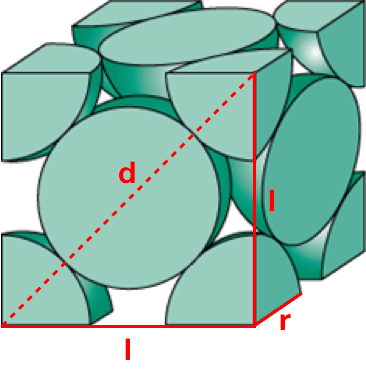# Problem: Platinum (atomic radius = 1.38 Å) crystallizes in a cubic closely packed structure. Calculate the edge length of the face-centered cubic unit cell and the density of platinum.

###### FREE Expert Solution

Recall: The face-centered cubic cell contains an atom in each of the face of the cube and an atom in each of the corners. It looks like this:Edge Length: If r = radius of the sphere, then the diagonal d = 4r, since it spans through 2 spheres. We can express the length of the cube l in terms r, using Pythagorean theorem.

88% (43 ratings)###### Problem Details

Platinum (atomic radius = 1.38 Å) crystallizes in a cubic closely packed structure. Calculate the edge length of the face-centered cubic unit cell and the density of platinum.